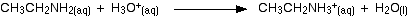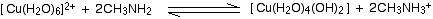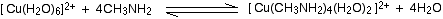# Amines as Bases

$$\newcommand{\vecs}{\overset { \rightharpoonup} {\mathbf{#1}} }$$ $$\newcommand{\vecd}{\overset{-\!-\!\rightharpoonup}{\vphantom{a}\smash {#1}}}$$$$\newcommand{\id}{\mathrm{id}}$$ $$\newcommand{\Span}{\mathrm{span}}$$ $$\newcommand{\kernel}{\mathrm{null}\,}$$ $$\newcommand{\range}{\mathrm{range}\,}$$ $$\newcommand{\RealPart}{\mathrm{Re}}$$ $$\newcommand{\ImaginaryPart}{\mathrm{Im}}$$ $$\newcommand{\Argument}{\mathrm{Arg}}$$ $$\newcommand{\norm}{\| #1 \|}$$ $$\newcommand{\inner}{\langle #1, #2 \rangle}$$ $$\newcommand{\Span}{\mathrm{span}}$$ $$\newcommand{\id}{\mathrm{id}}$$ $$\newcommand{\Span}{\mathrm{span}}$$ $$\newcommand{\kernel}{\mathrm{null}\,}$$ $$\newcommand{\range}{\mathrm{range}\,}$$ $$\newcommand{\RealPart}{\mathrm{Re}}$$ $$\newcommand{\ImaginaryPart}{\mathrm{Im}}$$ $$\newcommand{\Argument}{\mathrm{Arg}}$$ $$\newcommand{\norm}{\| #1 \|}$$ $$\newcommand{\inner}{\langle #1, #2 \rangle}$$ $$\newcommand{\Span}{\mathrm{span}}$$

We are going to have to use two different definitions of the term "base" in this page. A base is

• a substance which combines with hydrogen ions. This is the Bronsted-Lowry theory.
• an electron pair donor. This is the Lewis theory.

The easiest way of looking at the basic properties of amines is to think of an amine as a modified ammonia molecule. In an amine, one or more of the hydrogen atoms in ammonia has been replaced by a hydrocarbon group. Replacing the hydrogens still leaves the lone pair on the nitrogen unchanged - and it is the lone pair on the nitrogen that gives ammonia its basic properties. Amines will therefore behave much the same as ammonia in all cases where the lone pair is involved.

## The reactions of amines with acids

These are most easily considered using the Bronsted-Lowry theory of acids and bases - the base is a hydrogen ion acceptor. We'll do a straight comparison between amines and the familiar ammonia reactions. Ammonia reacts with acids to produce ammonium ions. The ammonia molecule picks up a hydrogen ion from the acid and attaches it to the lone pair on the nitrogen.If the reaction is in solution in water (using a dilute acid), the ammonia takes a hydrogen ion (a proton) from a hydroxonium ion. (Remember that hydrogen ions present in solutions of acids in water are carried on water molecules as hydroxonium ions, H3O+.)

$NH_3 (aq) + H_3O^+ (aq) \rightarrow NH_4^+ + H_2O (l)$

If the acid was hydrochloric acid, for example, you would end up with a solution containing ammonium chloride - the chloride ions, of course, coming from the hydrochloric acid. You could also write this last equation as:

$NH_3 (aq) + H^+ \rightarrow NH_4^+ (aq)$

. . . but if you do it this way, you must include the state symbols. If you write H+ on its own, it implies an unattached hydrogen ion - a proton. Such things don't exist on their own in solution in water. If the reaction is happening in the gas state, the ammonia accepts a proton directly from the hydrogen chloride:

$NH_3 (aq) + HCl (g) \rightarrow NH_4^+ (s) + Cl^- (s)$

This time you produce clouds of white solid ammonium chloride.

The nitrogen lone pair behaves exactly the same. The fact that one (or more) of the hydrogens in the ammonia has been replaced by a hydrocarbon group makes no difference.

Example 1: Ethylamine

If the reaction is done in solution, the amine takes a hydrogen ion from a hydroxonium ion and forms an ethylammonium ion.Or:The solution would contain ethylammonium chloride or sulfate or whatever. Alternatively, the amine will react with hydrogen chloride in the gas state to produce the same sort of white smoke as ammonia did - but this time of ethylammonium chloride.

$CH_3CH_2NH_2 (g) + HCl(g) \rightarrow CH_3CH_2NH_3^+ (s) + Cl^- (s)$

These examples have involved a primary amine, but it makes no real difference if a secondary or tertiary amine were used.; the equations would just look more complicated. The product ions from diethylamine and triethylamine would be diethylammonium ions and triethylammonium ions respectively.## The Reactions of Amines with Water

Again, it is easiest to use the Bronsted-Lowry theory and, again, it is useful to do a straight comparison with ammonia. Ammonia is a weak base and takes a hydrogen ion from a water molecule to produce ammonium ions and hydroxide ions. However, the ammonia is only a weak base, and doesn't hang on to the hydrogen ion very successfully. The reaction is reversible, with the great majority of the ammonia at any one time present as free ammonia rather than ammonium ions.

$NH_3 (aq) + H_2O (l) \rightleftharpoons NH_4^+ (aq) + OH^- (aq)$

The presence of the hydroxide ions from this reaction makes the solution alkaline. The amine still contains the nitrogen lone pair, and does exactly the same thing. For example, with ethylamine, you get ethylammonium ions and hydroxide ions produced.

$CH_3CH_2NH_2 (aq) + H_2O (l) \rightleftharpoons CH_3CH_2NH_3^+ (aq) + OH^- (aq)$

There is, however, a difference in the position of equilibrium. Amines are usually stronger bases than ammonia (there are exceptions to this, though - particularly if the amine group is attached directly to a benzene ring).

## The reactions of amines with copper(II) ions

Just like ammonia, amines react with copper(II) ions in two separate stages. In the first step, we can go on using the Bronsted-Lowry theory (that a base is a hydrogen ion acceptor). The second stage of the reaction can only be explained in terms of the Lewis theory (that a base is an electron pair donor).

Example 2: Reaction between Ammonia and Copper (II)

Copper(II) sulphate solution, for example, contains the blue hexaaquacopper(II) complex ion -$$[Cu(H_2O)_6]^{2+}$$.

In the first stage of the reaction, the ammonia acts as a Bronsted-Lowry base. With a small amount of ammonia solution, hydrogen ions are pulled off two water molecules in the hexaaqua ion.

This produces a neutral complex - one carrying no charge. If you remove two positively charged hydrogen ions from a 2+ ion, then obviously there isn't going to be any charge left on the ion. Because of the lack of charge, the neutral complex isn't soluble in water, and so you get a pale blue precipitate.

$[Cu(H_2O)_6]^{2+} + 2NH_3 \rightleftharpoons [Cu(H_2O)_4(OH)_2] + 2NH_4^+$

This precipitate is often written as Cu(OH)2 and called copper(II) hydroxide. The reaction is reversible because ammonia is only a weak base. That precipitate dissolves if you add an excess of ammonia solution, giving a deep blue solution.

The ammonia replaces four of the water molecules around the copper to give tetraamminediaquacopper(II) ions. The ammonia uses its lone pair to form a co-ordinate covalent bond (dative covalent bond) with the copper. It is acting as an electron pair donor - a Lewis base.

$[Cu(H_2O)_6]^{2+} + 4NH_3 \rightleftharpoons [Cu(NH_3)_4(H_2O)_2]^{2+} + 4H_2O$

The color changes are:## The corresponding reaction with amines

The small primary amines behave in exactly the same way as ammonia. There will, however, be slight differences in the shades of blue that you get during the reactions.

Example 3: Reaction between Methylamine and Copper (II)

With a small amount of methylamine solution you will get a pale blue precipitate of the same neutral complex as with ammonia. All that is happening is that the methylamine is pulling hydrogen ions off the attached water molecules.With more methylamine solution the precipitate redissolves to give a deep blue solution - just as in the ammonia case. The amine replaces four of the water molecules around the copper.As the amines get bigger and more bulky, the formula of the final product may change - simply because it is impossible to fit four large amine molecules and two water molecules around the copper atom.

## Contributors

Amines as Bases is shared under a not declared license and was authored, remixed, and/or curated by Jim Clark.